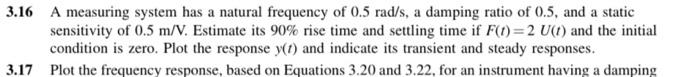# Question Solved1 Answer3.16 A measuring system has a natural frequency of 0.5 rad/s, a damping ratio of 0.5, and a static sensitivity of 0.5 m/V. Estimate its 90% rise time and settling time if F(t)=2 U() and the initial condition is zero. Plot the response y(t) and indicate its transient and steady responses. 3.17 Plot the frequency response, based on Equations 3.20 and 3.22, for an instrument having a dampingXMIBCU The Asker · Mechanical EngineeringTranscribed Image Text: 3.16 A measuring system has a natural frequency of 0.5 rad/s, a damping ratio of 0.5, and a static sensitivity of 0.5 m/V. Estimate its 90% rise time and settling time if F(t)=2 U() and the initial condition is zero. Plot the response y(t) and indicate its transient and steady responses. 3.17 Plot the frequency response, based on Equations 3.20 and 3.22, for an instrument having a damping
More
Transcribed Image Text: 3.16 A measuring system has a natural frequency of 0.5 rad/s, a damping ratio of 0.5, and a static sensitivity of 0.5 m/V. Estimate its 90% rise time and settling time if F(t)=2 U() and the initial condition is zero. Plot the response y(t) and indicate its transient and steady responses. 3.17 Plot the frequency response, based on Equations 3.20 and 3.22, for an instrument having a damping